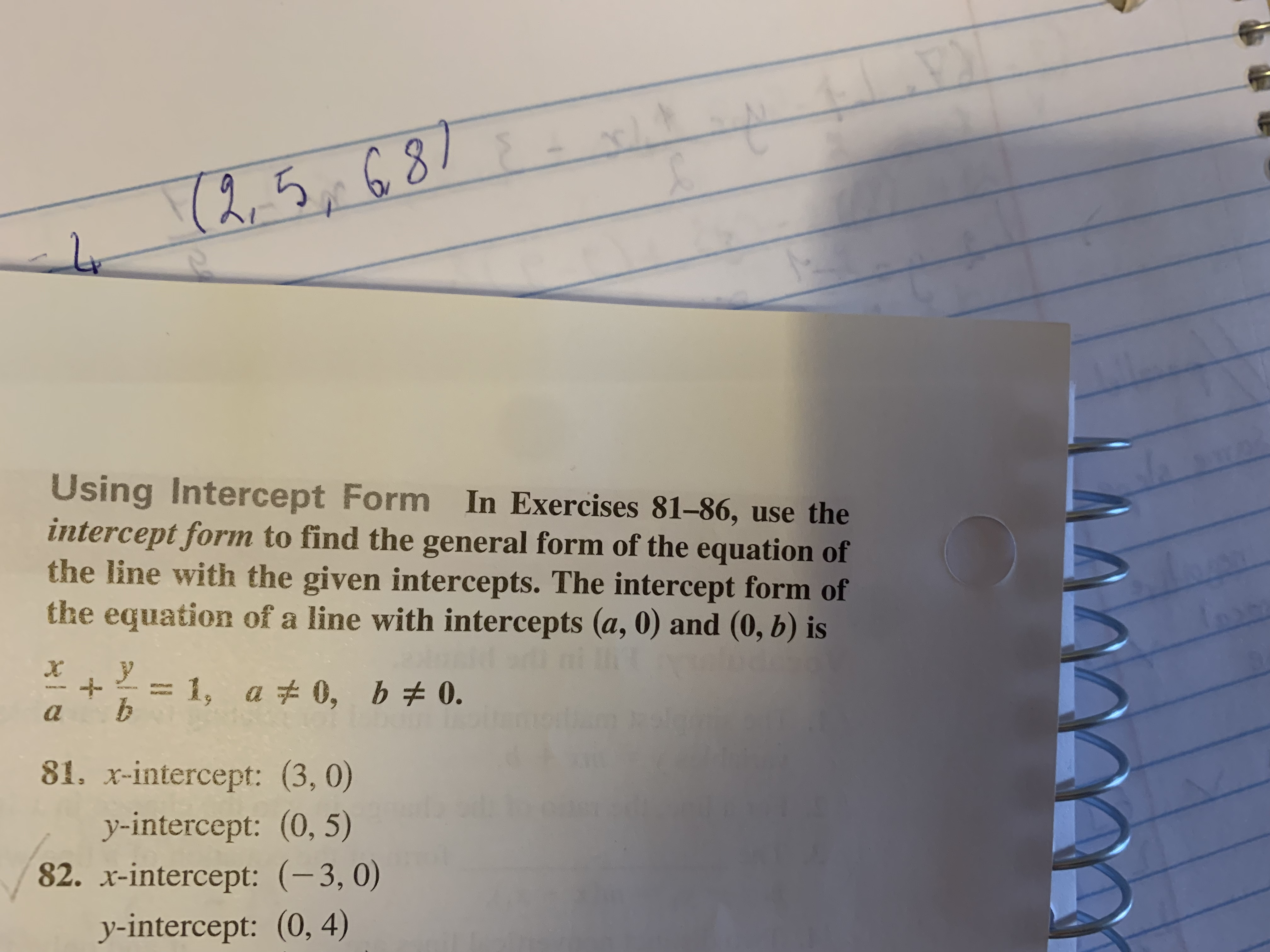# (2.53 Using Intercept Form In Exercises 81-86, use the intercept form to find the general form of the equation of the line with the given intercepts. The intercept form of the equation of a line with intercepts (a, 0) and (0, b) is da 81. x-intercept: (3, 0) y-intercept: (0, 5) 82. r-intercept: (-3, 0) y-intercept: (0, 4)

Question

And this one but only 82help_outlineImage Transcriptionclose(2.53 Using Intercept Form In Exercises 81-86, use the intercept form to find the general form of the equation of the line with the given intercepts. The intercept form of the equation of a line with intercepts (a, 0) and (0, b) is da 81. x-intercept: (3, 0) y-intercept: (0, 5) 82. r-intercept: (-3, 0) y-intercept: (0, 4) fullscreen

### Want to see this answer and more?

Experts are waiting 24/7 to provide step-by-step solutions in as fast as 30 minutes!*